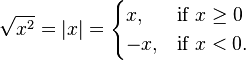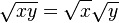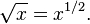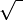# Square Root Code in Java : Simple Gui with Example

Before discussing  square root code in java , we should understand the term square root first .

square root of a number n is a number x such that x2 = n or , a number x whose square
is n .

There is also a built in method in java to calculate square root that is in java.math package which has sqrt() method.

The square root of x is rational if and only if x is a rational number that can be represented as a ratio of two perfect squares. ( square root of 2 is an irrational number, and quadratic irrational  for all non-square natural numbers.) The square root function maps rational numbers into algebraic numbers (a superset of the rational numbers).
For all real numbers x$square root swing example$
For all non-negative real numbers x and y,$square root gui example$
and$square root example in java$

Square roots of negative numbers are not real numbers – they are imaginary numbers. Every complex number except 0 has 2 square roots. For example: −1 has two square roots. We call them$square root code in java$ and$square root java$.
The sign for a square root is made by putting a bent line over a number, like this:$square root in java$. We say "the square root of 4" (or whatever number we are taking the square root of).
A whole number with a square root that is also a whole number is called a perfect square. The first few perfect squares are: 0, 1, 4, 9, 16, 25, 36, 49, 64, 81, 100, 121, 144, 169, 196, 225...

Symbol :

## It is not really known where the square root symbol$square root program in java$ comes from, but some people believe that it was from the letter r, which is the first letter of the Latin and German wordradix. Radix means square root.

Let us understand how java built in square root method works :

Square root code in java in java.lang.math  class :

```    public static double sqrt(double a) {
return StrictMath.sqrt(a); // default impl. delegates to StrictMath
// Note that hardware sqrt instructions
// frequently can be directly used by JITs
// and should be much faster than doing
// Math.sqrt in software.
}
```

in StrictMath java file it is implemented like this

```    /**
* @param   a   a value.
* @return  the positive square root of {@code a}.
*/
public static native double sqrt(double a);
```

So in precise words , square root code in java  is implemented in machine language  depending upon  the operating system .

Demo :

Square root code in java :

```import java.awt.Button;
import java.awt.Frame;
import java.awt.Label;
import java.awt.TextField;
import java.awt.event.ActionEvent;
import java.awt.event.ActionListener;
import java.awt.event.KeyEvent;
import java.awt.event.KeyListener;

public class SquareRoot implements ActionListener,KeyListener
{
Frame f;
Label one,two;
TextField three,four;
Button five;
Double i,t;
String s1="";
public SquareRoot()
{
f=new Frame("square root");
one=new Label("Enter the number");
two=new Label("square root is ");
three=new TextField(5);
four=new TextField(5);
five=new Button("Ckick here for result");
f.setSize(400,400);
f.setVisible(true);
f.setLayout(null);
one.setBounds(20,20,140,40);
two.setBounds(20,250,140,40);
three.setBounds(180,30,140,40);
four.setBounds(180,250,140,40);
five.setBounds(100,150,240,40);
}
public void keyPressed(KeyEvent k)
{
System.out.print("");
}
public void keyTyped(KeyEvent k)
{
s1+= k.getKeyChar();
}
public void keyReleased(KeyEvent k)
{
System.out.print("");
}
public void actionPerformed(ActionEvent ae)
{

try
{
t=Double.parseDouble(s1); // this is another method to convert string into double

// Float f=Float.valueOf(s1);  //Returns the Float object that contains the value specified by the string in str.
// Float f1=f.floatValue();    //Returns the value of the invoking object as a float.
// System.out.print(f1);

}
catch(NumberFormatException e)
{
}

for(i=0.01;i<=t;)
{
System.out.println((double)(i*i));

if(((float)(i*i)/t==.99)||(float)(i*i)/t==1.0)
{
four.setText(i+"");
System.out.print("                  "+"subham"+i);
break;
}

i=i+.01;

}
}
public static void main(String s[])
{
new SquareRoot();
}
}
```

References : Oracle docs for Math class in lang package